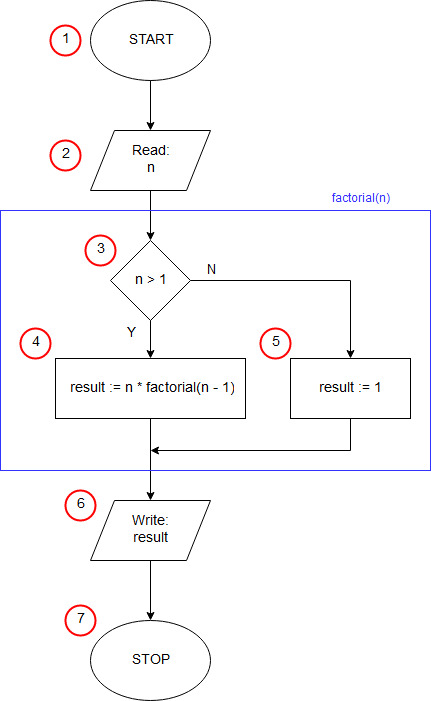# Factorial in Pascal - recursive

<<algorithmprogram Fact_rec; function factorial(n: integer): integer; begin if n>1 then factorial:=n*factorial(n-1) else factorial:=1; end; var n, f: integer; begin write('n = '); readln(n); f:=factorial(n); writeln(n,'! = ',f); readln; end.

The first line of the program can be treated as an ornament, but required by the syntax of the language pascal. Every program in the pascal must start with the keyword program preceding the name of the program, it adds nothing to the implementation of the algorithm.
In the third line, we declare a function called factorial, taking one integer parameter (n) and returning a whole number as a result. Between the keywords begin with lines 4 and end of lines 9, there is a function body - a set of instructions executed by the program after calling this function.
In line 5 we check if n is greater than 1 - block (3) of the algorithm. If so, in line 6, we determine the result of the function as n multiplied by the value of the function factorial (the same function as the one we currently define) for n minus 1 (4). At this point, the function factorial calls itself. This function is called a recursive function. If n is not greater than 1, then in line 8 we set the result of the function equal to 1 (recursive termination condition) - block (5).
Before starting the operational part of the program on line 11, we declare two integer variables (variables n and the result). In the pascal, all variables must be declared before the block of code starts. When declaring a variable, we must specify what type of data will be stored in it. Declaration of variables starts with the keyword var.
12 the line containing the keyword begin corresponds to the starting block (1) of our algorithm.
Lines 13 and 14 perform the input/output operation (2), in which the input data is taken - the number n, whose factorial we want to calculate.
In the line 15, the result variable is assigned the result of the function factorial called with the argument n.
On line 16, we print the result obtained (input-output block (6)).
The readln statement on line 17 has nothing to do with the execution of the algorithm. It serves to stop the program until you press the enter key, which prevents the program window from closing immediately.
Instruction end. from line 18, the program ends (end block of the algorithm (7)).